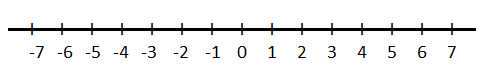QUESTION

# Which of the following options is correct for the following blank in the statement below ?Every point on a number line represents _ _ .A. A natural numberB. A rational numberC. A unique numberD. A irrational number

Hint: Let us draw a number line and then check which type of numbers line on the number line whether they are natural, rational, irrational or irrational.

As we know that there are infinitely many points in a number line which we cannot even plot. So, some of the major points in the number line are plotted below.As we know from the definition of natural numbers that natural numbers are those which are used for counting and ordering or we can say that natural numbers are the positive integers starting from 1 and goes to infinity.
Some of the natural numbers are 1, 2, 3, 4.
As we know that in a number line natural numbers lie but all points do not represent natural numbers because the number line also has natural numbers.
Now as we know that according to the definition of rational number any number is known as rational number if it is expressed as quotient or fraction $\dfrac{p}{q}$ of two integers where p is the numerator and q is the denominator. Since p and q are integers. So, q can also be equal to 1. So, every integer is a rational number.
Like $2,{\text{ }} - 5,{\text{ }}0,{\text{ }}\dfrac{{ - 6}}{5},{\text{ }} - \dfrac{{71}}{9}$ are some of the rational numbers.
In the number some points represent rational numbers but there are infinitely many points. So, all points do not represent rational numbers.
And according to the definition of irrational number any number is known as irrational if it is not rational or in other words we can say that the number cannot expressed as quotient or fraction $\dfrac{p}{q}$ of two integers where p is the numerator and q is the denominator and both are integers.
Like $\pi ,{\text{ }}0.0001010011010....$ are some of the irrational numbers.
In the number line there are some points that represent irrational numbers, but all points do not represent irrational numbers because natural numbers are also present in the number line and natural numbers are not irrational.
And unique means different. So, unique numbers are different numbers. So, no two of them are the same.
But we can clearly say that all points in a number line represent a different number either it is a rational, irrational or natural number. But it is unique.
So, every point in a number line represents a unique number.
Hence, the correct option will be C.

Note:- Whenever we come up with this type of problem then first we should plot a number line and then we should take note that every type of a number occurs in a number line because as name suggests that it is a number line either number is rational, irrational, natural or a real it occurs in number line. But if we say that each point represents which type of number then we cannot answer anyone because each point does not represent the same type of number. But all numbers are unique. So, each point must represent a unique number.